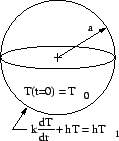## Examples, Green's Function Numbers

In this section several examples are given of numbering system designations. The Green's function number depends on the body shape and the types of boundaries present. The temperature number, also given, includes additional information on the boundary conditions, the initial conditions, and the volume energy generation (if present).

Rectangular coordinate examples.
1.
Slab with specified heat flux at x = 0 and specified temperature at x = L. Initial temperature is T0.
The GF number is X21.
The temperature number is X21B11T1.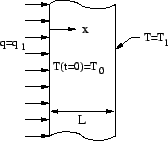2.
Slab with insulated boundary at x = 0 and specified temperature at x = L. Initial temperature is T1.
The GF number is X21.
The temperature number is X21B01T1.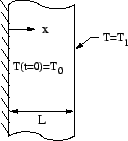3.
Rectangle with specified temperature at x = 0, convection at x = a, time-varying heat flux at y = 0, and, specified temperature at y = b. The initial temperature is zero.
The GF number is X13Y21.
The temperature number is X13B01T0Y21B21.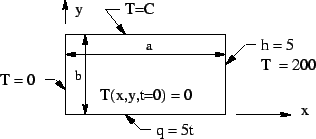4.
Semi-infinite body which is heated non-uniformly over its surface. The initial temperature is uniform.
The GF number is X20Y00.
The temperature number is X20B-T1Y00.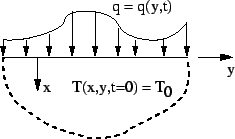Cylindrical coordinate examples.
1.
Solid cylinder with time-varying exponential heat flux at r = a and with initial temperature of T0.
The GF number is R02.
The temperature number is R02B4T1.2.
Semi-infinite cylinder heated uniformly over a circular region and insulated elsewhere on its surface. The initial temperature is zero.
The GF number is R02X20.
The temperature number is R02B0T0 X20B(r5).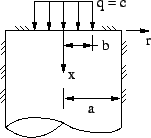Spherical coordinate example.
1.
Solid sphere with convection at radius r = a and with spatially uniform internal energy generation. The initial temperature is T0.
The GF number is RS03.
The temperature number is RS03B1T1G1.